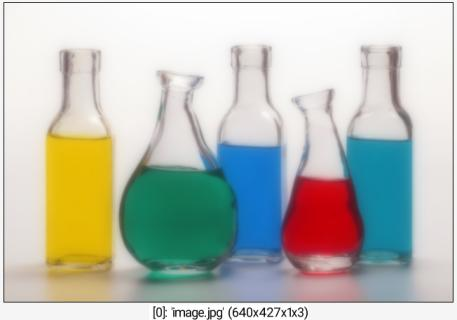G'MIC - GREYC's Magic for Image Computing: A Full-Featured Open-Source Framework for Image ProcessingReference

# blur_bloom

## Arguments:

• _amplitude>=0,_ratio>=0,_nb_iter>=0,_blend_operator={ + | max | min },_kernel={ 0=deriche | 1=gaussian | 2=box | 3=triangle | 4=quadratic },_normalize_scales={ 0 | 1 },_axes

## Description:

Apply a bloom filter that blend multiple blur filters of different radii,

resulting in a larger but sharper glare than a simple blur.
When specified, argument axes is a sequence of { x | y | z | c }.
Specifying one axis multiple times apply also the blur multiple times.
Reference: Masaki Kawase, "Practical Implementation of High Dynamic Range Rendering", GDC 2004.

## Default values:

amplitude=1, ratio=2, nb_iter=5, blend_operator=+, kernel=1, normalize_scales=0 and axes=(all)

## Example of use:

\$ gmic image.jpg blur_bloom ,Command: image.jpg blur_bloom ,
G'MIC - GREYC's Magic for Image Computing: A Full-Featured Open-Source Framework for Image Processing